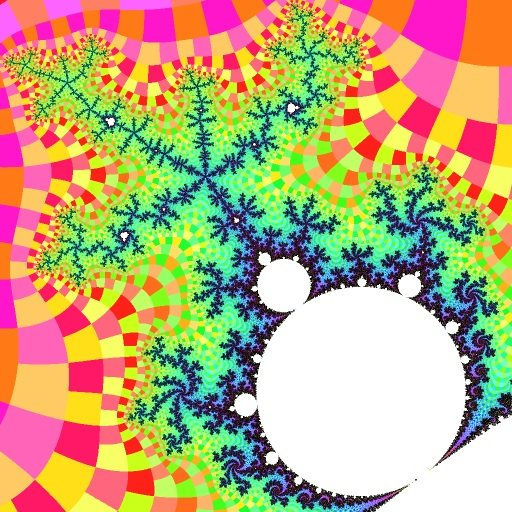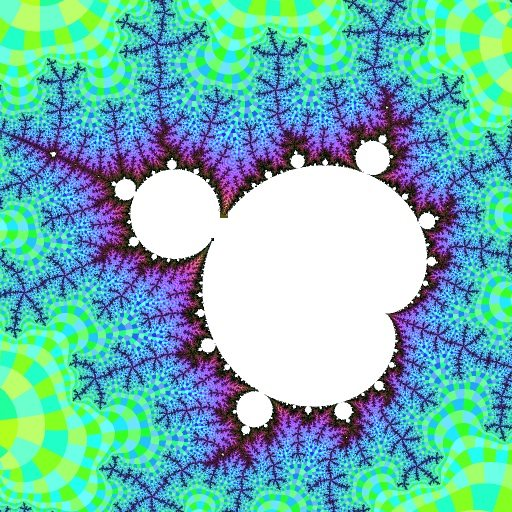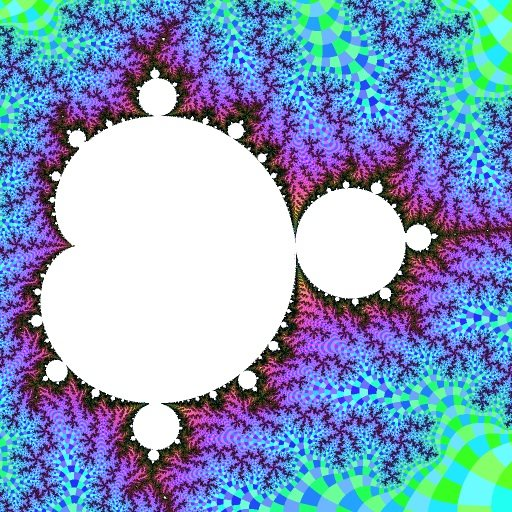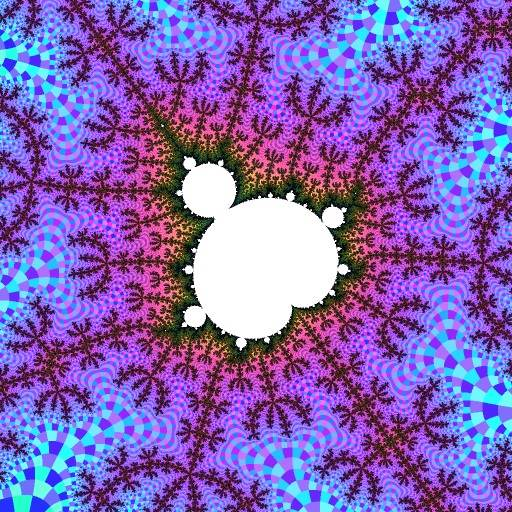mdbtxt1
mdbtxt2
Proceed to Safety

# R2.2/5a

Robert P. Munafo, 2008 Feb 1.

R2.2/5a is the R2-name for the largest period-5 mu-atom, and R2.2/5 is its mu-unit.

The "northwest radical" is sometimes used to refer to R2.2/5 (or "northwest bulb" for R2.2/5a), however the northwest external angle goes to a completely different mu-unit, R2.1/2.1/3.

Here is a picture of R2.2/5 and the attached filaments R2F(2/5B) :-0.5434 + 0.6132 i @ 0.19 -.. R2F(2/5B2) R2F(2/5B1) '`T-._ , .:\_ .,_,'\$.r", __- ._e" -- .d^^ . '`^\-"(``-/"c -`'"--,Ca* '@__e , .@"_ _.a~'ve,_ .. '^^\;, :_.,-Ye,%(Y_ /(` .r)LaaC`_._ ._(v_ -rdd"*L/*"/` -dY-~"\$"`., ''\$d, ._./@` ^^F` `` :/ ^Fmba- 'Y@` d-@"@T"- '~""Fv_/@.d*F- "` .:e .,._.@TC\, :e_ .:"@r . ___(,-am*"F/ 'dYL,brc, :,.m- '^"bc.\$F`__, vv`, .'^LF-*mbF" :vd@Fd"*`,'*@/@m_ee v*Y@/@d\$CCm,mmL _d^C..,"d^e\ ^ ^ *@/TF@_Yb-/mdC^^* _ "^**^@_^"YmF*` -,m-T"-"%b)^'" _., .me\$F*YdbFbmde,'m@md`a_m_dY@"a_ ^ """`:%r ^^^` '*dCc.^]@@` 'T@c:mem@@\d%@@*Y@YF-__,._ '' ..C)FdF@@; .@d**"^-'*""Y...@Y" @Fb@C R2F(2/5B3) '^\db -@@Ce,__ '' ^^Y"@_/%\$Fbmc '` .d"`%/@*` '"""@@`'` _/e ._, _d@\d@C` '"@~@b[ ^*bm\$C\, -*^@C^` "@@"* _.eL"@% .:dc]d; 'Y@@- ., _\$rd@*`__"@F**; R2.2/5a .Y@c .a :a@L/`-@^(@Y\@@L,._ .@"_md@ ^ `_\$*m,'*FFv@(\$@c a/\m*^^ '@\7` _.e_/^@@_ .(-^ '^@@Fadb_m,, -` mF*@%*^@%YCa_,, _, ^ ^\$YF^bd"@bmmeervm"^ '' '` '^`
View: -0.5434 +0.6132i @ +0.1900
R2.2/5a and R2F(2/5B*)

Three of the filaments have been labeled, they are R2F(2/5Bn) for n={1,2,3}. Each of these filaments has a pretty-good sized island mu-molecule located near its midpoint, and the three islands are all about the same size, which makes this view particularly attractive (see also R2.1/2F(1/3B1)S).

R2F(2/5B1)S, R2F(2/5B2)S, and R2F(2/5B3)S are the 12th, 10th and 13th-largest (respectively) islands in the whole Mandelbrot Set, and R2F(2/5(1/2B1)B1)S is the 20th largest. Here they are, all shown at exactly 32 times the scale of the above picture:-0.597865 + 0.663411 i @ 0.005938 R2F(2/5B1)S (the 12th largest island)-0.529367 + 0.668227 i @ 0.005938 R2F(2/5B2)S (the 10th largest island)-0.592316 + 0.620091 i @ 0.005938 R2F(2/5B3)S (the 13th largest island)-0.550769 + 0.626543 i @ 0.005938 R2.2/5F(1/2B1)S (the 20th largest island)

### The Island Clusters Search

The islands in R2F(2/5(*B)) have a special property: they form a "cluster" of similarly-sized islands.

To search for other places in the Mandelbrot set that have this property, one can examine all secondary continental mu-units, searching in order of the denominator of their internal angle.

For each one, examine the surrounding area to find all attached island mu-molecules, and mesure their areas. I compare the area of the largest such island to the total area of the others.

In such a search, R2F(2/5(*B)) sets a record; the record is set again by R2F(2/7(*B)), then by R2F(5/12(*B)), R2F(3/19(*B)), R2F(3/22(*B)), R2F(3/25(*B)), R2F(3/28(*B)), and R2F(3/31(*B)), the last five being of the form R2F(3/3N+1(*B)).

The first two are of the form R2F(2/2N+1(*B)); other promising patterns include R2F(5/5N+3(*B)), R2F(7/7N+2(*B)), R2F(12/12N+5(*B)), R2F(12/12N+7(*B)) and R2F(3N+1/6N+5(*B)).

Other record-setters that don't fit one of those patterns include R2F(7/33(*B)), R2F(19/45(*B)), R2F(17/46(*B)), R2F(19/50(*B)), R2F(8/51(*B)), R2F(23/51(*B)), R2F(19/60(*B)), R2F(17/63(*B)), R2F(19/64(*B)), and R2F(23/64(*B)) .

revisions: 20080201 oldest on record; 20100918 improve image and formatting; 20100921 add colloquial names; 20101021 add ranks of 4 largest islands

From the Mandelbrot Set Glossary and Encyclopedia, by Robert Munafo, (c) 1987-2022.     Mu-ency index

This page was written in the "embarrassingly readable" markup language RHTF, and was last updated on 2010 Oct 21.s.27